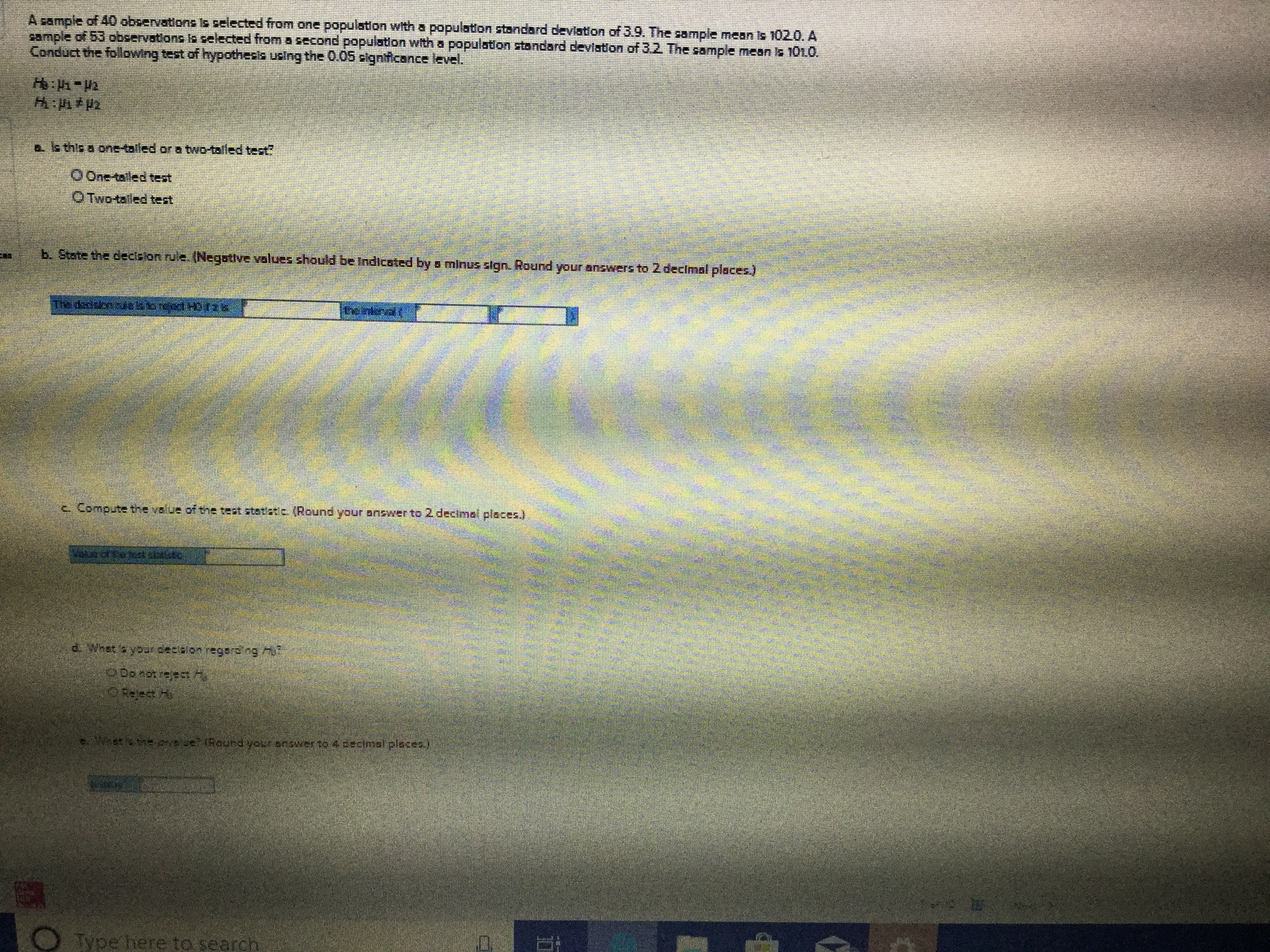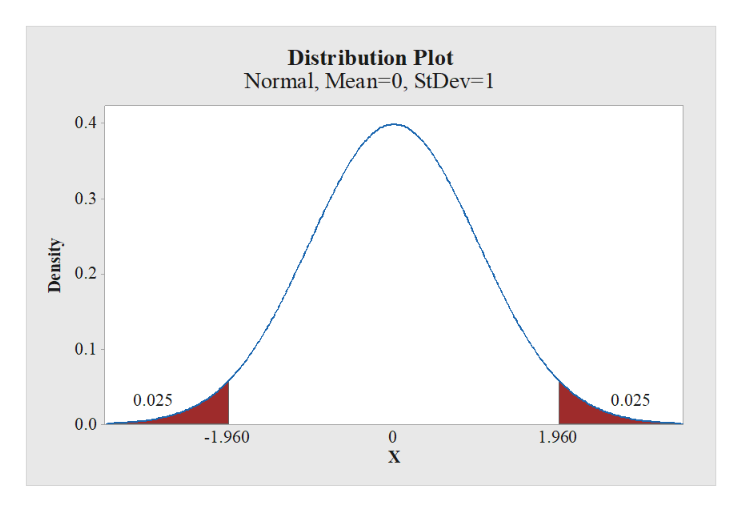# A sample of 40 observationsls selected from one population with a population standard deviation of 3.9. The sample mean is 102.0. Acample of 53 observstions Ioelected from8second popuiation with a population stondard deviaton of 3.2. The sample mean is 1010Conduct the followng test of hypothesis ucing th 0.05 pignficance level.trt-d:a is this a one-tailed or a two-taled test?OOne-taled testOTwo-taled testb. State the decslon ru'e. (Negative volues should be Indicated by s minus slgn. Round your answers to 2 decimal places)కా గజివtc Compute tne value ofthe teat statatc (Round your Bnswer to 2 decimal places)·ఆ9: శng 7:PDonorrojecetstheesei (Rourdyour anower to 4cecimal plocea)Type here to sear

Question
15 views

I would like the solution for each part of the problemhelp_outlineImage TranscriptioncloseA sample of 40 observationsls selected from one population with a population standard deviation of 3.9. The sample mean is 102.0. A cample of 53 observstions Ioelected from8second popuiation with a population stondard deviaton of 3.2. The sample mean is 1010 Conduct the followng test of hypothesis ucing th 0.05 pignficance level. trt-d: a is this a one-tailed or a two-taled test? OOne-taled test OTwo-taled test b. State the decslon ru'e. (Negative volues should be Indicated by s minus slgn. Round your answers to 2 decimal places) కా గజివt c Compute tne value ofthe teat statatc (Round your Bnswer to 2 decimal places) · ఆ9: శng 7: PDonorrojec etstheesei (Rourdyour anower to 4cecimal plocea) Type here to sear fullscreen
check_circle

Step 1

Hey, since there are multiple subparts posted, we will answer first four question. If you want any specific question to be answered then please submit that question only or specify the question number in your message.

(a)

The alternative hypothesis state no direction. Moreover, “≠” symbol is in alternative hypothesis. Hence, the test is two-tailed test.

(b)

Step-by-step procedure to obtain the value of z using MINITAB:

• Choose Graph > Probability Distribution Plot choose View Probability > OK.
• From Distribution, choose ‘Normal’ distribution.
• Choose Probability and Both Tail for the region of the curve to shade.
• Enter the Probability value as 05.
• Click OK.

Output using the MINITAB software is given below:help_outlineImage TranscriptioncloseDistribution Plot Normal, Mean 0, StDev=1 0.4 0.3 0.2 0.1 0.025 0.025 0.0 -1.960 1.960 0 X Density fullscreen
Step 2

From the output, the critical value is ±1.96.

The decision rule is,

If z<–1.96, then reject the null hypothesis H0 ...

### Want to see the full answer?

See Solution

#### Want to see this answer and more?

Solutions are written by subject experts who are available 24/7. Questions are typically answered within 1 hour.*

See Solution
*Response times may vary by subject and question.
Tagged in

### Hypothesis Testing